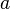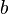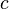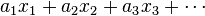# Coefficient facts for kids

Kids Encyclopedia Facts

In mathematics, a coefficient is a constant multiplicative factor of a certain object. For example, the coefficient in 9x2 is 9. A coefficient can also be a variable such as$a$,$b$ and$c$.

The object can be such things as a variable, a vector, a function, etc. In some cases, the objects and the coefficients are indexed similarly, leading to expressions such as:$a_1 x_1 + a_2 x_2 + a_3 x_3 + \cdots$

where an is the coefficient of the variable xn for each n = 1, 2, 3, …

## Related pagesCoefficient Facts for Kids. Kiddle Encyclopedia.